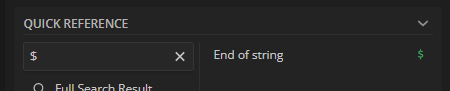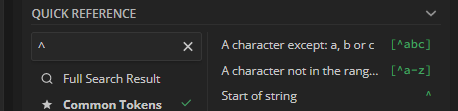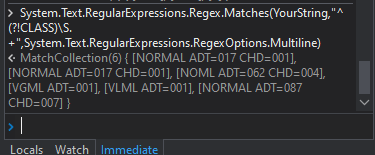# Regex problem : working on regexr.com but not in UI path

Hello !

I’m very happy to ask my first question… hope that you could help me.

## CLASS M ADT=151 CHD=011 INF=001

My objectives :
I must ignore CLASS… lines and ------------- lines.
I must scrap complete others lines (ex : NORMAL ADT=017 CHD=001 or VGML ADT=001)

I’ve created this REGEX which works on regexr.com
^(?!CLASS)((?=([a-zA-Z]{4,6}))(.*?)((?<=[a-zA-Z]{3}=[0-9]{3})\$|(?<=[a-zA-Z]{3}=[0-9]{3} [a-zA-Z]{3}=[0-9]{3})\$))

But when I used it in UI Path, it’s doesn’t works. Maybe it’s related to ^ and \$

My declaration on UI path
System.Text.RegularExpressions.Regex.Matches(txtLine,“(?!CLASS)((?=([a-zA-Z]{4,6}))(.*?)((?<=[a-zA-Z]{3}=[0-9a{3})\$|(?<=[a-zA-Z]{3}=[0-9]{3} [a-zA-Z]{3}=[0-9]{3})\$))”)

Thanks for all help !

Sorry for bold. I don’t know how to edit my original post

Hello,

In your UiPath expression you didn’t put ^ in first, is it desired ?

Here \$ will represent the End of the stringAlso ^ will represent the starting of the stringCan you share the sample input and Output

Regards
Gokul

Hi,

Can you try Multiline option as the following?

``````System.Text.RegularExpressions.Regex.Matches(txtLine,"^(?!CLASS)((?=([a-zA-Z]{4,6}))(.*?)((?<=[a-zA-Z]{3}=[0-9]{3})\$|(?<=[a-zA-Z]{3}=[0-9]{3} [a-zA-Z]{3}=[0-9]{3})\$))",System.Text.RegularExpressions.RegexOptions.Multiline)
``````

Regards,

Yes,

## CLASS M ADT=151 CHD=011 INF=001

My output on REGEXR :

So maybe in UI Path ^ and \$ must be used differently… because when I suppress them, regex works but not as I needed

I’m trying another approach, line by line… for exemple that one :

With this REGEX :
(?![CLASS])(?=[a-zA-Z]{4,6})(.*?)([a-zA-Z]{3}=[0-9]{3}|[a-zA-Z]{3}=[0-9]{3} [a-zA-Z]{3}=[0-9]{3})

On regexr, I get only :

I could have for each line NORMAL ADT=0xx CHD=0xx or NORMAL ADT=0xx
Problem come from OR operator, could you tell me I could use it ?
Thanks !

Maybe an alternate using String Manipulation can be done in the below way :

``````String.Join(Environment.NewLine,Split(strText,Environment.NewLine).Where(Function(x) Not(String.IsNullOrWhiteSpace(x) orElse x.Trim.ToUpper.StartsWith("CLASS"))))
``````

Let us know if you are still having issues or provide a feedback.

I’ve found a more simple solution …
-Split the file into an array
-Iterate on each line by using a more simple regex :
(?![CLASS])(?=([a-zA-Z]{4,6}))(.*?)([a-zA-Z]{3}=[0-9]{3})
-If this regex return results that’s means that I could apply my process : I don’t need to capture the complete line with my regex, for that, I could use the line object.

Hello @Florent_Delage make sure to use a .Net Regex Tester so your pattern would work on UiPath. Try this link: .NET Regex Tester - Regex Storm

Thank you for the link !

``````System.Text.RegularExpressions.Regex.Matches(YourString,"^(?!CLASS)\S.+",System.Text.RegularExpressions.RegexOptions.Multiline)